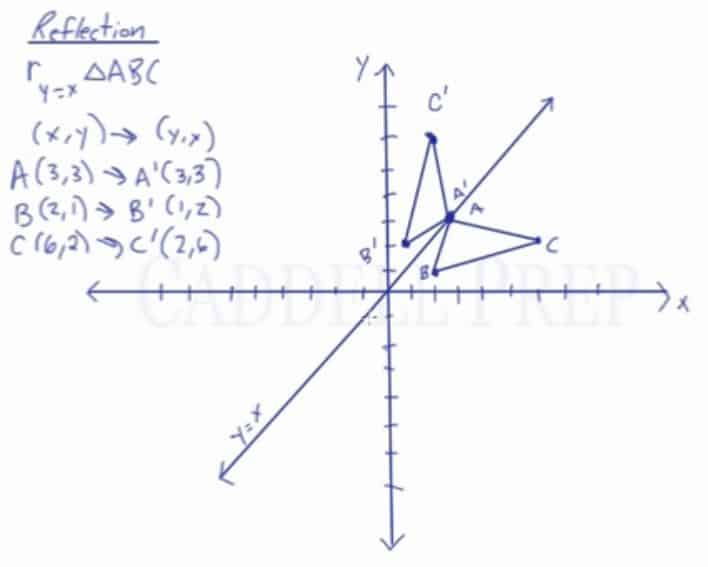In this video, you will learn how to do a reflection over the line y=x.

The line y=x, when graphed on a graphing calculator, would appear as a straight line cutting through the origin with a slope of 1.

When reflecting coordinate points of the pre-image over the line, the following notation can be used to determine the coordinate points of the image:
ry=x=(y,x)

For example:

For triangle ABC with coordinate points A(3,3), B(2,1), and C(6,2), apply a reflection over the line y=x.

By following the notation, we would swap the x-value and the y-value.
A(3,3), B(2,1), and C(6,2) would turn into
A'(3,3), B'(1,2), and C'(2,6)## Video-Lesson Transcript

In this lesson, we’ll take a look at reflecting over a line$y = x$.$r_{y = x}$

First of all, what is a line$y = x$?

This is a line that for every$x$ value, we get the same$y$ value.$y = x$ will look something like this. A diagonal straight line.

When$x = 1$,$y = 1$. When$x = 2$,$y = 2$. And when$x = 3$,$y = 3$. And so on.

If we have a point, for example,$(3, 1)$, we’re going to reflect it over. We need to move perpendicular to it.

One side should be perpendicular to the other side.

Our image now is$(1, 3)$.

All we did was switch the$x$ and the$y$ values.

For example, if the initial image is$(1, -4)$.

We have to make a line perpendicular to measure the distance. Then make the same line distance on to the other side.

The reflected point is$(-4, 1)$.

When we reflect over the line$y = x$, we just switch the values of$x$ and$y$.$r_{y = x} (x, y) \rightarrow (y, x)$

Let’s look at an example where we’ll reflect triangle ABC over the line$y = x$ using the coordinates.$r_{y = x} \triangle{ABC}$

We know that the rule is the coordinates$(x, y)$ is going to switch to$(y, x)$. We’ll simply switch them.$A (3, 3) \rightarrow A^\prime (3, 3)$$B (2, 1) \rightarrow B^\prime (1, 2)$$C (6, 2) \rightarrow C^\prime (2, 6)$

Let’s graph this now. And draw a triangle.

Now, we have a reflection of triangle$ABC$ over the line$y = x$ to form the image of$A^\prime B^\prime C^\prime$.